# Practice Problems: Confidence Intervals & Sample Size Confidence Intervals

 Date 01.02.2018 Size 21.9 Kb.
Practice Problems: Confidence Intervals & Sample Size

Confidence Intervals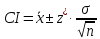or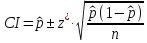1. A sample of Alzheimer's patients is tested to assess the amount of time in stage IV sleep. It has been hypothesized that individuals suffering from Alzheimer's Disease may spend less time per night in the deeper stages of sleep. Number of minutes spent is Stage IV sleep is recorded for sixty-one patients. The sample produced a mean of 48 minutes (= 14 minutes) of stage IV sleep over a 24 hour period of time. Compute a 95 percent confidence interval for this data. What does this information tell you about a particular individual's (an Alzheimer's patient) stage IV sleep?

1. A university wants to know more about the knowledge of students regarding international events. The are concerned that their students are uninformed in regards to new from other countries. A standardized test is used to assess student’s knowledge of world events (national reported mean = 65, = 5). A sample of 30 students are tested (sample mean = 58,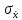= 3.2). Compute a 99 percent confidence interval based on this sample's data. How do these students compare to the national sample?

1. A sample of students from an introductory psychology class was polled regarding the number of hours they spent studying for the last exam. All students anonymously submitted the number of hours on a 3 by 5 card. There were 24 individuals in the one section of the course polled. The data was used to make inferences regarding the other students taking the course. There data are below:
 4.5 22 7 14.5 9 9 3.5 8 11 7.5 18 20 7.5 9 10.5 15 19 2.5 5 9 8.5 14 20 8

Compute a 95 percent confidence interval. What does this tell us?

Margin of Error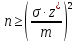or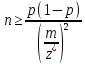1. How many patients would need to be used to estimate the success rate of medical procedure, if researchers initially believe the success rate is no smaller than 85% and wish to estimate the true success rate using a 95% confidence interval with a margin of error no larger than .03?

1. What sample size would be necessary to estimate the mean cholesterol level for the population of females between the ages of 30 – 40 with a 95% confidence interval that has a margin of error no larger than 5 mg/dl?

Probabilities and z-scores

1. A survey conducted to measure the height of American males. In the survey, respondents were grouped by age. In the 20 – 29 years old group, the heights were normally distributed with a mean of 69.2 inches and a standard deviation of 2.9 inches. If a random male from that group was chosen, what is the probability that his height would be greater than 72 inches?

1. The lengths of Atlantic croaker fish are normally distributed with a mean of 10 inches and a standard deviation of 2 inches. If one fish is chosen randomly, what is the probability that the length of that Atlantic croaker fish is between 7 and 15 inches?

1. In a recent year, the ACT scores for high school students with 3.5 – 4.0 GPA’s were normally distributed with a mean of 25.2 with a standard deviation of 4.6. If a sample of 25 students with 3.5 – 4.0 GPA’s were randomly chosen, what is the probability that the sample’s average ACT score would fall below 24?